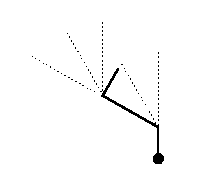# Internet Problem Solving Contest

## Problem F – Fractal Plants

The scientists from our university discovered a new kind of plant (in the corner of one long-forgotten storage room). The plant was interesting because it seemed to be totally useless. Still, the mathematician who discovered it quickly became fascinated by its structure.

The plant has no leaves and no branches, only one long and twisted stem. The stem is equally thick everywhere. Its exact shape is a very complicated curve defined by a genetic code stored in the seed. This code is interpreted when a plant is growing.

More exactly, suppose that we have a cartesian coordinate system with the x-axis going from left to right and the y-axis going upwards. The seed is planted at the point (0,0), the initial growth direction is upwards and the initial growth unit is 1 cm. Starting from the seed our plant grows by interpreting its genetic code in the following way:

• f – grow one growth unit in the current growth direction
• l – turn the growth direction 30 degrees left
• r – turn the growth direction 30 degrees right
• @{q} (where q is a rational number written in decimal notation) – multiply the growth unit by q
• (any other character) – do nothing

The following picture shows a plant with code fll@{2}frxyzrr@{0.5}fWhile growing, the plant's stem may cross itself and even bury itself under ground (i.e., have a part with a negative y coordinate). In other words, for all our needs the plant is just a general polyline in the given vertical plane.

After interpreting the whole code the plant is ripe, it produces a next generation seed and dies. The next generation seed has a more complex code stored in it. It is produced from the parent's code by replacing each letter of the original code by some given string. (All occurrences of the same letter are replaced by the same string, and the strings are the same for all generations of the plant.)

### Problem specification

Given the string w0 (the genetic code of the initial plant), a set of strings used to create the next generation code, and an integer K, determine the coordinates of the center of mass of a K-th generation plant. (The initial plant is considered to be a 0-th generation plant.)

### Input specification

The first line of the input file contains an integer T specifying the number of test cases. Each test case is preceded by a blank line.

The first line of each test case contains two integers K (the number of generations) and L (the number of defined strings). The second line contains the initial genetic code w0. L lines follow, each of them defines the replacement string for one letter. Each of these lines has the format a:s(a), where a is a letter and s(a) is the string used to replace all occurrences of the letter a when forming the code for the next generation of the plant. (If for some letter a the value s(a) is not defined in the input, we assume that s(a)=a.)

### Output specification

For each test case output a single line containing the x and y coordinates of the center of mass of the given plant. We accept any output with precision error up to 10-7. (Be sure to specify enough decimal places for both x and y!)

### Example

Input:

```2

117 2
xf
x:x@{0.5}
f:ff

1 2
xf
x:x@{0.333333333333333}
f:fllfrrrrfllf
```

Output:

```0 0.5
-0.072168784 0.5
```

Note:
The generations of the second plant look more and more like the Koch curve.

Credits:
Problemsetter(s): misof, Tom
Contest-related materials: Tom, Palo